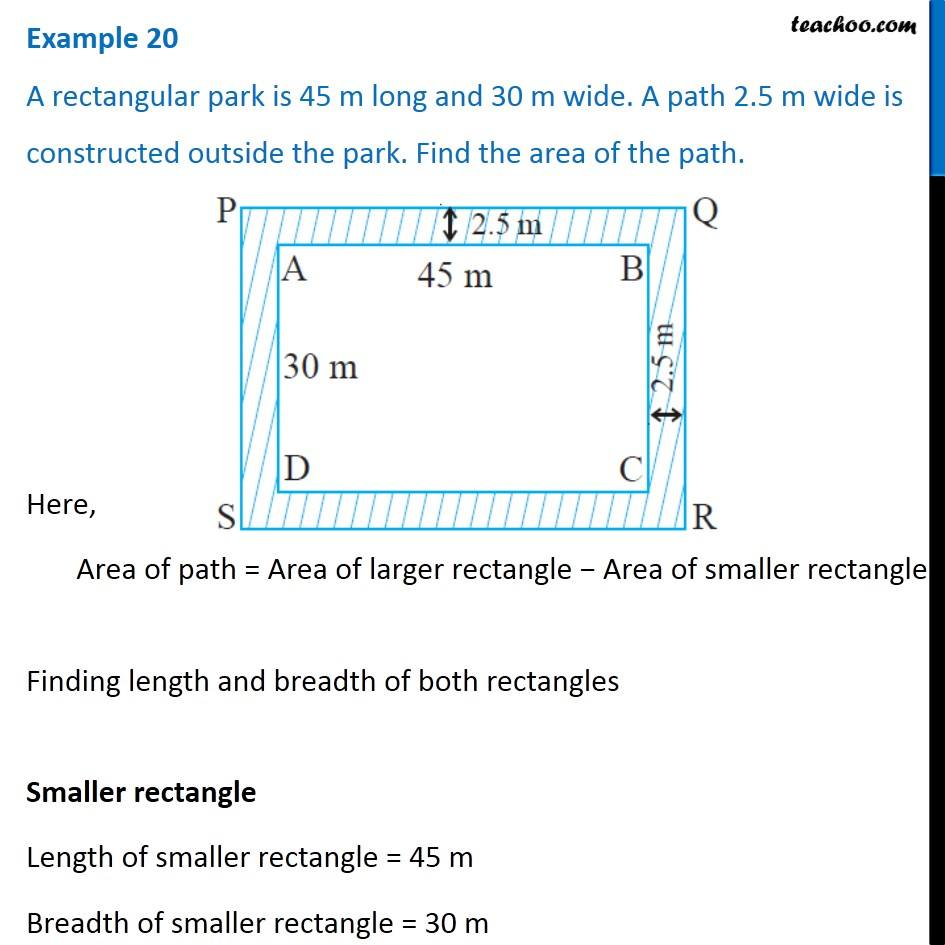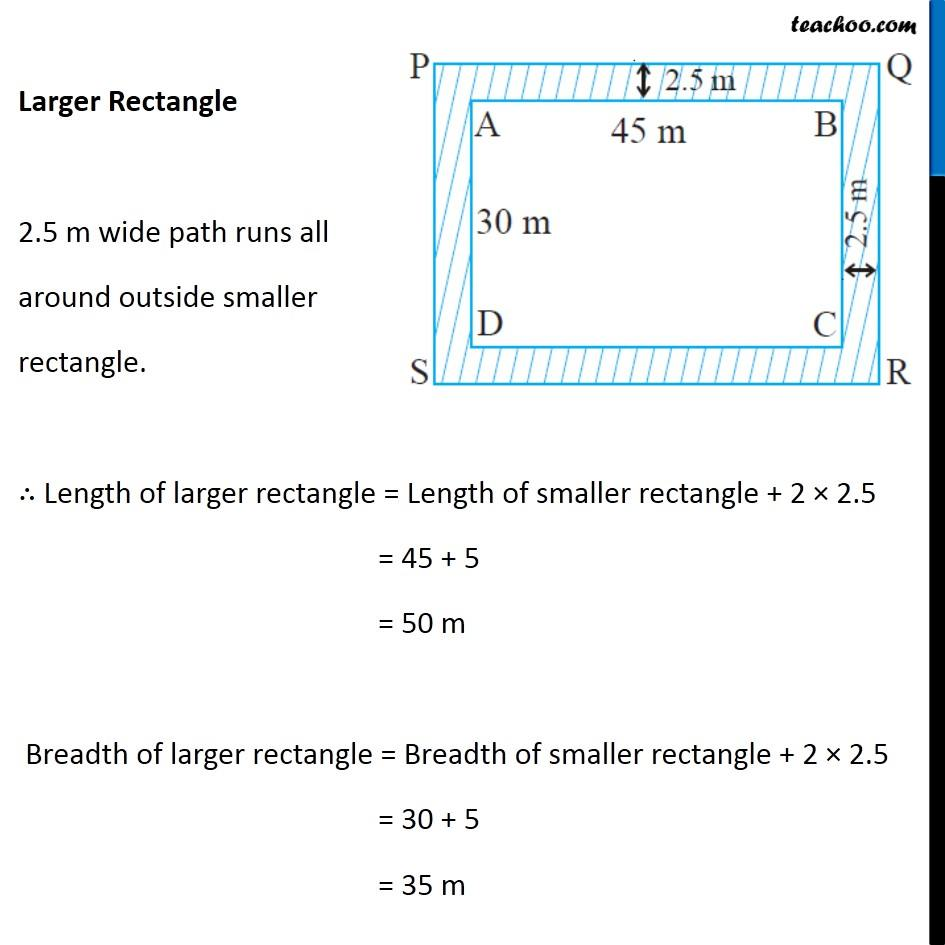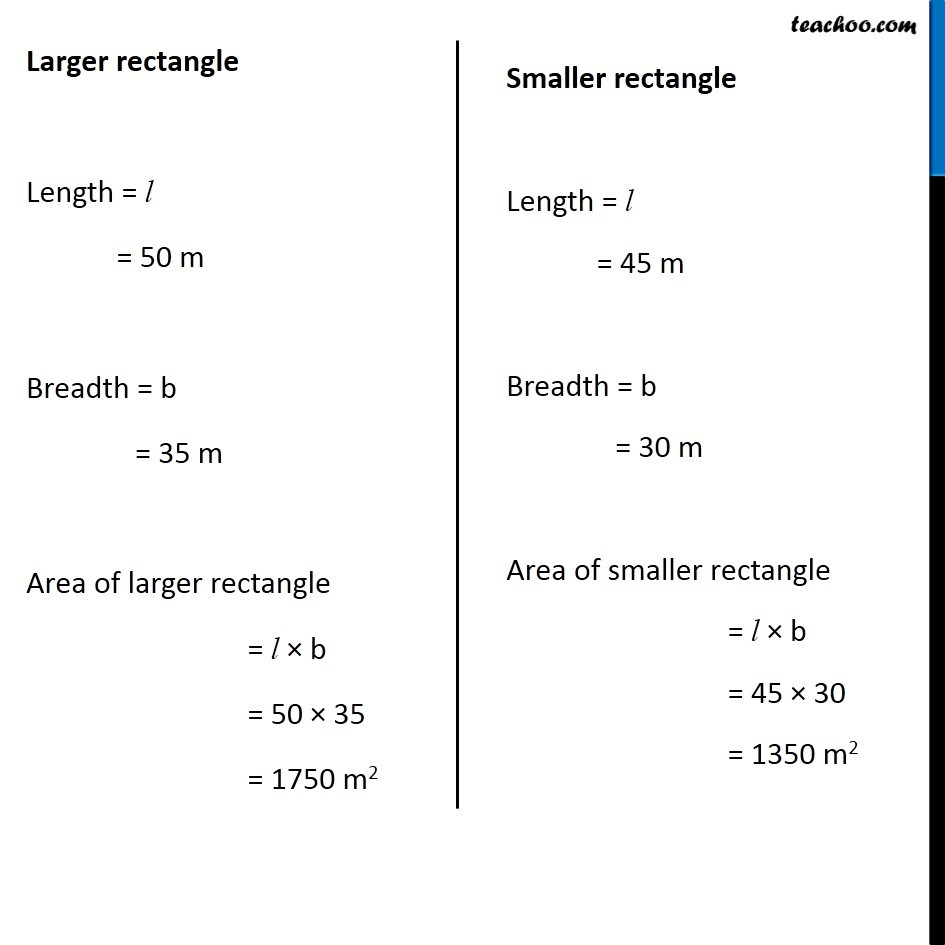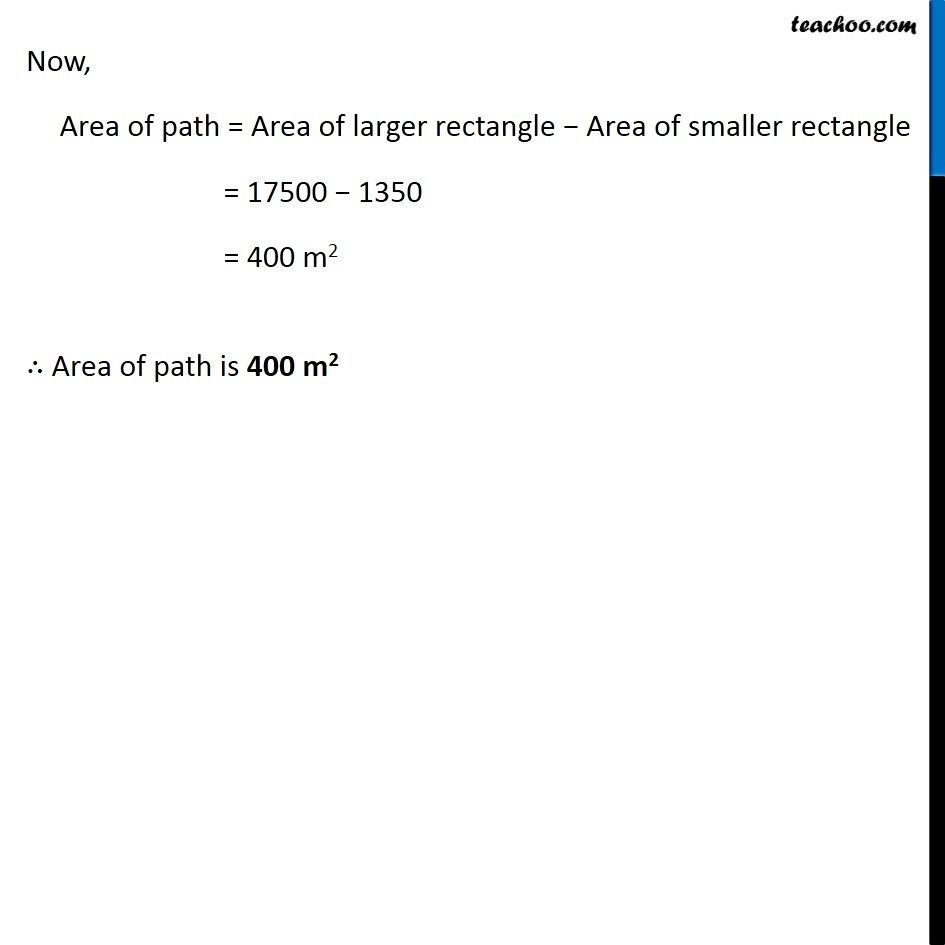1. Chapter 11 Class 7 Perimeter and Area
2. Concept wise
3. Finding Area of rectangle with path outside/inside

Transcript

Example 20 A rectangular park is 45 m long and 30 m wide. A path 2.5 m wide is constructed outside the park. Find the area of the path. Here, Area of path = Area of larger rectangle − Area of smaller rectangle Finding length and breadth of both rectangles Smaller rectangle Length of smaller rectangle = 45 m Breadth of smaller rectangle = 30 m Larger Rectangle 2.5 m wide path runs all around outside smaller rectangle. ∴ Length of larger rectangle = Length of smaller rectangle + 2 × 2.5 = 45 + 5 = 50 m Breadth of larger rectangle = Breadth of smaller rectangle + 2 × 2.5 = 30 + 5 = 35 m Larger rectangle Length = l = 50 m Breadth = b = 35 m Area of larger rectangle = l × b = 50 × 35 = 1750 m2 Smaller rectangle Length = l = 45 m Breadth = b = 30 m Area of smaller rectangle = l × b = 45 × 30 = 1350 m2 Now, Area of path = Area of larger rectangle − Area of smaller rectangle = 17500 − 1350 = 400 m2 ∴ Area of path is 400 m2

Finding Area of rectangle with path outside/inside

About the AuthorDavneet Singh
Davneet Singh is a graduate from Indian Institute of Technology, Kanpur. He has been teaching from the past 10 years. He provides courses for Maths and Science at Teachoo.